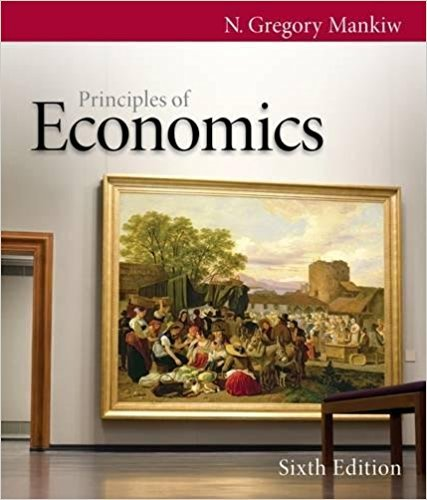×

×

# Solutions for Chapter 25: Production and Growth## Full solutions for Principles of Economics | 6th Edition

ISBN: 9780538453059Solutions for Chapter 25: Production and Growth

Solutions for Chapter 25
4 5 0 407 Reviews
30
2
##### ISBN: 9780538453059

Principles of Economics was written by and is associated to the ISBN: 9780538453059. Chapter 25: Production and Growth includes 18 full step-by-step solutions. Since 18 problems in chapter 25: Production and Growth have been answered, more than 61301 students have viewed full step-by-step solutions from this chapter. This expansive textbook survival guide covers the following chapters and their solutions. This textbook survival guide was created for the textbook: Principles of Economics, edition: 6.

Key Business Terms and definitions covered in this textbook

the ability to produce a good using fewer inputs than another producer

the ability to produce a good using fewer inputs than another producer

• average variable cost

variable cost divided by the quantity of output

• constant returns to scale

The property whereby long-run average total cost stays the same as the quantity of output changes

• consumer surplus

the amount a buyer is willing to pay for a good minus the amount the buyer actually pays for it

• efficiency

the property of society getting the most it can from its scarce resources

• efficient scale

the quantity of output that minimizes average total cost

• exports

goods produced domestically and sold abroad

• income effect

the change in consumption that results when a price change moves the consumer to a higher or lower indifference curve

• inflation rate

the percentage change in the price index from the preceding period

• internalizing the externality

altering incentives so that people take account of the external effects of their actions

• marginal product of labor

the increase in the amount of output from an additional unit of labor

• normal good

a good for which, other things being equal, an increase in

• proportional tax

a tax for which highincome and low-income taxpayers pay the same fraction of income

• quantity equation

the equation M × V = P × Y, which relates the quantity of money, the velocity of money, and the dollar value of the economy’s output of goods and services

• quantity supplied

the amount of a good that sellers are willing and able to sell

• shortage

a situation in which quantity demanded is greater than quantity supplied

• total revenue (for a firm)

the amount a firm receives for the sale of its output

• utility

a measure of happiness or satisfaction

• vertical equity

the idea that taxpayers with a greater ability to pay taxes should pay larger amounts